Shockwave Calculator:

Introduction:
This applet calculates the property variations across a normal or oblique shockwave under two sets of assumptions. The first choice is the standard assumption of a calorically (and thermally) perfect gas. The gas can be any that meets the assumptions provided The gas constant and specific heat ratio are known. The second option is for standard equilibrium air at densities from 1,000 amagats to 1e-7 amagats and temperatures up to 25,000 K. This analysis accounts for real gas effects of a standard mixture of species in air including dissociation and ionization. This analysis is generally quite accurate for values within the stated density range, but can only be used with air. The program provides many options for specifying upstream properties and provides quick calculation. The output can be summarized to be copied and pasted into a word processor, and the applet provides a platform-independent, easy-to-use interface.
Acknowledgements:
I would like to thank Dr. William Devenport and Dr. Bernard Grossman, as they are the people who have written a very important part of this code. Although the shock algorithms and interfacing have been coded by me, the real gas effects algorithm has been coded by them, with the original coding work done by Dr. Grossman in FORTRAN. The curve fit tables for this algorithm comes from . The source code for this file is quite complex, so I would like to thank them for something which would be very difficult to reproduce! More of their similar work can be found at www.engapplets.vt.edu.
Typical Steps for Solution:
1. Select the shocktype, either normal or oblique, and under either real or calorically perfect assumptions.
2. Select units as convenient.
3. Specify exactly 2 known upstream properties by typing their values into the upstream boxes, and select those two by selecting the checkboxes appropriate to each. If the analysis is calorically perfect, the user has the option of entering no upstream properties instead, but, in this case, the downstream ratios button is automatically selected.
4. If the analysis is of an oblique shockwave, enter an angle in the appropriate box and select that angle by checking the checkbox. For a calorically perfect oblique shock, either theta or beta can be entered. For a real oblique shock, only theta is accepted.
5. If the analysis is calorically perfect, make sure that R and gamma are correct, or change their values to fit the gas being analyzed.
6. Enter either an upstream mach number or upstream velocity and select the checkbox next to it.
7. decide if either the ratios of downstream to upstream or the downstream stagnation values are desired and check those boxes as appropriate.
8. That's it!! Hit the "Calculate" button for the solution in the right hand column!
Users Guide: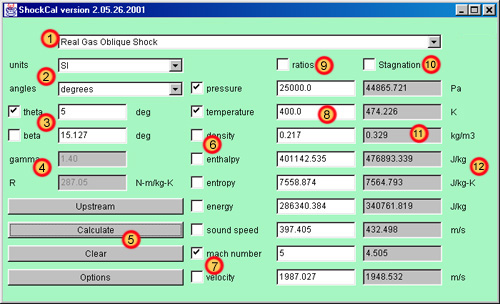1. Shock Type Selection Choice - This menu allows the uer to choose from four different types of shockwaves. For the assumptions and applicability of each type of shockwave, see the technical theory section below.
2. Units Choice - Choices are provided for property units in either SI or British systems of measurement and angles in either degrees or radians. The boxes of property and angle values are succeeded by the specific appropriate units, which will change depending on the choices made here. Of course, make sure that all values given to the program are given in the units asked for.
3. Angle Specification - the angle specification is used only for oblique shocks (both calorically perfect and real). Selecting a normal shock will gray out this area. Most typically, theta is known, but one of the two angles must be known and specified to carry out this analysis. Note that the real gas oblique shock can ONLY accept theta as an input parameter (it is unlikely that beta would be known anyway). The calorically perfect oblique shock accepts either. Indicate which is the known quantity by checking the appropriate checkbox. The other angle that is calculated in the analysis is filled in by the program as part of the solution.
4. Constants Specification - The specific heat ratio and gas constant are used only for the calorically perfect shocks. Selecting a real shock will gray out this area. The default values are appropriate for standard air at modest temperatures. These parameters must be specified for other gases. (see the technical theory section below for information on how to get these numbers for other cases).
5. Action Buttons - Four buttons are present which controls the actions of the program.
 Upstream: This button calculates all upstream properties for either a real or calorically perfect gas. Two properties must be specified by the user in order to calculate the rest. Acceptable choice of the two specified properties are defined in the technical theory. If a mach number or velocity is specified, the code will also calculate the other upstream value. Otherwise, this is left blank. Entering invalid data will generate an error message. Calculate: This button causes all upstream data to be calculated as in the button above. Then, the changes across the shockwave are calculated and displayed. If a real gas shockwave, two upstream properties, and either mach or velocity must be specified. If a calorically perfect gas shockwave, the same is true, except that only upstream mach number can be specified if desired (output is automatically only the property ratios however). Also, gamma and R must be given in this case. If the analysis is an oblique shockwave, an angle must also be given. Clear: This button clears all enteered data and resets the screen for a new analysis. Options: This button brings up a dialog box giving some options for the program. These options include how many decimal places to output (internally, all values are double precision), and the option of showing a results summary window of each shock which a user can copy and paste to a text editor.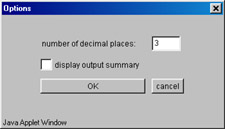6. Upstream Property Selection - The checkboxes are for letting the program know which two thermodynamic quantities the user has specified. The code will read these two, and calculate the rest when the Upstream or Calculate buttons are pressed. The code requires exactly two (and bars a user from entering more). Not entering a valid number generates an error message. For calorically perfect shocks, no properties can be entered, but this forces the code to generate property ratios only. The technical theory section lists acceptable property choices for all types of shocks.
7. Speed Selection - For calculating the changes across the shock, either velocity or mach number must be specified upstream. Mach number must be specified for the calorically perfect shock with no upstream properties. The checkbox indicates to the code which is specified. Not checking one box or not entering a valid number next to the checked item results in an error.
8. Upstream Properties/Speeds - These are the fields for entering the upstream properties. Of course, valid numbers must be entered. Entering data in a box that is not checked will be ignored by the program, and exactly 2 properties and one speed must be given for calculation. The code fills in the rest of the values.
9. Ratio Checkbox - This option tells the program to output the ratio of DownStr./UpStr. instead of the actual downstream values. Very often, the ratios of downstream to upstream are more informative than the actual values. This can be combined with the Stagnation checkbox (below).
10. Stagnation Checkbox - This option tells the program to output the value of the stagnation properties rather than static values. Stagnation properties are described in the technical theory section. This can be combined with the ratio checkbox to ouput the ratio of downstrean stagnation properties to upstream stagnation value(i.e. Po2/Po1, To2/To1, etc.).
11. Downstream Properties/Speeds - These are the fields in which the results of the calculations are displayed. They are not editable (although the are copyable). Selecting the ratios checkbox makes them the ratio of downstream to upstream properties. Selecting the stagnation checkbox gives the stagnation properties downstream.
12. Units - These are the units of the properties, which are updated depending on the choice of units. These units apply to both upstream and downstream, unless the ratios checkbox is selected, in which case, downstream ratios are dimensionless.
Technical Theory:

Only a brief technical theory highlighting important points is presented here. Most of the theory used in this applet is documented in well-established sources, complete with derivations and equations.

First, the difference between a real and a calorically perfect gas is discussed. A calorically perfect gas is one in which the specific heats of the gas are constant. This is the relationship between the amount of energy in a gas to its temperature. A calorically perfect gas is one in which the specific heats do not vary as temperature increases or decreases. This is usually a good assumption for diatomic gases at moderate temperatures (around room temperature, or 300K). At significantly higher temperatures, the gas tends to dissociate and eventually ionize. Monatomic gases behave similarly, except that they can't dissociate, so calorically perfect validity tend to hold to much higher temperatures. If unsure, a good way to get a "feel" for the validity of the calorically perfect assumption is to try both real and calorically perfect normal shocks and compare answers. By raising the upstream mach number in increments, an analyst will see that at low mach numbers, both methods give similar downstream temperatures. As mach number increases, one will see that the calorically perfect assumption begins to predict temperatures much higher than for the real gas. This discrepency continues to grow with mach number.

The real gas effects are modeled by curve fit equations from  as coded by Dr. Grossman and Dr. Devenport(see above). These curve fits are quite involved and a reader is referred to that paper for more information on the details of the fits. The equations are valid for 1,000 to 1e-7 amagats and less than 25,000 K.

For the calorically perfect gas, the gas constant(R) and specific heat ratio(gamma) must always be specified. For air, the correct values are 1.40 for gamma, and 287.05 J/kg-K or 1716.20 lbf-ft/slug-R for R. These two numbers can be easily found for other gases as follows(from ): for any calorically perfect gas, gamma can be assumed to be 7/5 for any diatomic gas, and 5/3 for any monatomic gas. Also, R can be found by taking the universal gas constant, 8314 J/kgmol-K, and dividing by the molecular weight of the gas in question.

The calorically perfect formulation is the standard formulation given in many compressible flow books.  is a particularly good book and is recommended to learn the mechanics of this program. The calorically perfect shockwaves are solved exactly as outlined in chapters 3 and 4. Normal shocks are solved directly using the formulas for pressure, temperature, and density ratios given in chapter 3, which are functions only of mach number and gamma. Energy and enthalpy are obtained by the calorically perfect assumptions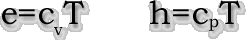Also, there is no analytical solution given for the absolute value of entropy given for a calorically perfect gas, except if based on a reference point. The label for entropy changes to "entropy change" for a calorically perfect analysis, and the upstream value is set to the reference value. The downstream solution is actually the entropy change, as given: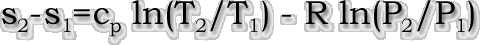For the oblique shock, first the derivative of the famous beta-theta-mach equation (see , chapter 4) is solved to find the maximum allowed theta, and corresponding beta are found. If the input theta is greater than theta_max, the shock is detached rather than oblique. This forms a complex flow field beyond the abilities of this program. Near the detachment point, a detached shock is more closely represented by a normal shock. Provided theta is less than theta_max, the beta-theta-mach equation is solved using the bisection method, and the normal upstream and downstream values are found as shown in .

For the real gas solutions, most of the algorithms are iterative, solving the general jump conditions for a normal and oblique discontinuity by the bisection method . This makes the solution of real gas equations more complicated and less reliable. For the oblique shock, only theta is allowed as an input, and since there is no analytical solution like the beta-theta-mach relation, the code runs a calorically perfect solution first to estimate whether theta exceeds theta_max. If this happens, the program will simply give erratic results and a warning is displayed. Also, through the iterative process, if the algorithm detects a point out of range of the real gas curve fits, it prompts the user with a warning.  and  give a great deal of background information on the handling and effects of real gases.

A stagnation property is defined as "what the value would be if the fluid was brought isentropically to a halt under the current circumstances." For stagnation properties in the calorically perfect case, the stagnation pressure, temperature, and densities are again calculated from the formulas of . Stagnation enthalpy and energy ratios are equal to the stagnation temperature ratio. Note that the stagnation temperature ratio is actually calculated even though it should always be 1. This is done as a check on the program. For both real and calorically perfect gases, by definition, static and stagnation entropy is identical!

For real gases, the stagnation enthalpy is calculated by definition from the jump conditions of a general fluid as follows: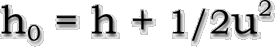and the attribute that stagnation properties are defined isentropically. Using the calculated stagnation enthalpy, and entropy from the solution, the real gas algorithm calculates the rest of the properties. By definition in both cases, velocity is zero.

The program (except under the ratio option of calorically perfect gases) requires exactly 2 upstream properties and and upstream mach number or speed to calculate -- plus and angle for oblique shocks. For the calorically perfect gas, acceptable parameter pairs are:
 pressure temperature pressure density temperature density density enthalpy density energy pressure enthalpy pressure energy
For the real gas air tables, acceptable property pairs are as follows:
 pressure temperature pressure density density energy entropy enthalpy pressure entropy
Tips for Use:
• because of the extreme narrowness of the solution, the iterative algorithm for the real oblique shock doesn't work well for M1 less than 1.5 or so. For 1 < M1 <1.75 or so, use the calorically perfect oblique shock instead. It is capable of solving down virtually to M1 = 1, and low mach numbers are the cases when the calorically perfect assumptions hold the best.
• for a real oblique shock, the beta-theta-mach equation is solved for a calorically perfect case in order to determine if the maximum theta has been exceeded and the shock is detached. If this happens, the real oblique shock will still provide whatever is gets, but a warning is displayed, and the solution is probably not valid at all.
• when in doubt, try a shock solution as both real and calorically perfect, and compare answers. In general, the higher the incoming mach number, the larger the discrepency.
• MAKE SURE THE UNITS MATCH WHAT THE PROGRAM IS ASKING FOR(Including RADIANS vs. DEGREES)!!!
• across all non-moving shocks, the stagnation enthalpy should remain the same. Therefore, selecting "ratios" and "stagnation", the downstream enthalpy reading should be very close to 1 (within a little numerical rounding). The same is true for stagnation temperature for calorically perfect gases. This is a good check that the program executed correctly.
Release Notes:
 version # build # changes/updates 2 06.13.2001 first version posted on WWW. 2 06.21.2001 fixed output window to not show units when the downstream values are ratios. fixed error message to list the acceptable properties for solution. 2 10.21.2001 some minor miscillaneous bugs in real gas subroutines fixed by W. Devenport.
References:
 Srinivasan S, Tannehill J C and Weilmuenster K J. "Simplified Curve Fits for the Thermodynamic Properties of Equilibrium Air", NASA RP1181, August 1987.
 Anderson, John. Modern Compressible Flow. 2nd ed. ISBN#0-07-001673-9. McGraw-Hill, 1990.
 Grossman, Bernard. "Fundamental Concepts of Real Gasdynamics". Virginia Tech/AOE department. Class notes, AOE5114, High Speed Aerodynamics. Version 3.09, January 2000.
 Vincenti, W.G. and Kruger, C.H. Introduction to Physical Gas Dynamics. New York: Wiley,1965.
 Liepmann, H.W. and Roshko, A. Elements of Real Gasdynamics. New York: Wiley, 1957.